MATH 383 at UNC. Fuck diff eq. Just fuck it.

```Studying for Diff Eq final

4.2

Solutions of nth order linear homogenous differential equations are of the form Z(r) = a_0(r^n)+a_1(r^n-1)+…a_n-1*r+a_n).

That is, simply, when a_0*r^n  + a_1*r^n-1  +…+a_n = 0, then you know that e^rt is a solution.
We know that this polynomial has n zeros, some of which may be equal.

If the roots are real, and no two are equal, then we have n distinct solutions. These n are e^r_1, e^r_2, etc. If they are linearly independent, then the general solution is y=c_1*e^r_1t + c_2*e^r_2*t, etc.

So if we have an equation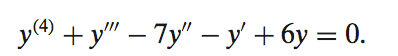then we put it in “r” form ￼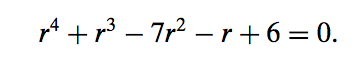and then solve for r (good luck, you can try eyeballing it, looking at the factors of the term with no r’s, or doing long division if you can partially factor it), you should get four solutions. And then we know the general solution is of the form ￼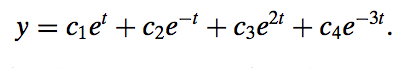Trick for figuring out roots of high order equations: look at ratios of factors of a_0 and a_n, so look at factors of the first coefficient and the last coefficient, and then take the ratio of latter over the former. You should do plus/minus for each value. Try that for a possible r, and repeat until you find a few. If you’ve found as many as n, then you’re done. If you haven’t, then divide the polynomial by the roots you found (so like long divide (r-3) into r^4+r^3…etc).

—————————————————————————

For complex solutions, that is, where you have a characteristic equation that has complex roots. You must first factor it as far as you can and then find the complex solutions. For example:Means that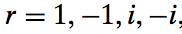We then need to use our handy equation￼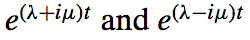= ￼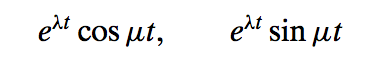. To figure out what we should put for the i and -i solutions. We get that they should be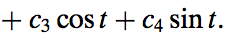———————————————————————————

For an equation where you have repeated roots, that is something like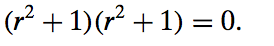which has solutions i,i, -i, and -i. Then you have to solve it by multiplying each of the repeated roots by t. So say you had three i’s, then you would need to do cos(t)+t*cos(t)+t^2*cos(t).

4.3

We do undetermined coefficients similarly to how we did before, but we have to be aware that the multiplicity of a root (how many times it exists in the solution, so like if you have (r-1)^3, you have a multiplicity of 3 in the equation) can be higher than 2 now.

For undetermined coefficients, we find the general solution in the normal way, but then we have to find a particular solution.

For the particular solution, we look at the solutions to the equation (e^t, t*e^t, etc) and choose a solution of the farm Y(t)=At^n+1*e^t where n is the highest degree solution??? (is this really the case, idk).  So given our guess of this form, Y(t), then we can use professor’s method, or differentiate Y(t) three times, and then substitute y and its derivatives, and set it equal to the right hand side or something. Solve for all the undetermined coefficients. Need to look over method of undetermined coefficients. Maybe go to office hours.

When we an equation that’s not homogeneous (one that has a few terms on the right hand side), we can write the solution as a sum of the solutions for each individual term. So for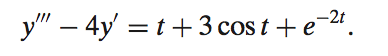, we solve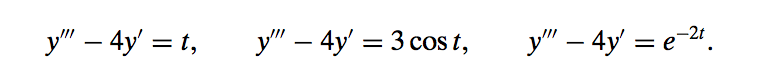.

So essentially we can boil things down to this: if not homogenous, first proceed like it is. once you come to a solution (based on the characteristic equation), you split up all of the terms on the right hand side and solve each of them using undetermined coefficients, which goes as follows: guess the solution form for the equation (ex: At+B), if a term in the solution form has the same degree of t as a term in the general solution, then we need to multiply this solution form by “t” until no terms are represented in the homogeneous solution. Now, one thing you need to do is talk to him about getting better at solving these undetermined coefficient problems. After you know how to do the solutions for A, B, etc, then you should be able to do the entire problem.

4.4

```